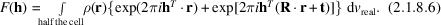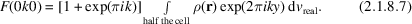International
Tables for
Crystallography
Volume F
Crystallography of biological macromolecules
Edited by E. Arnold, D. M. Himmel and M. G. Rossmann

International Tables for Crystallography (2012). Vol. F, ch. 2.1, pp. 60-61   | 1 | 2 |

## Section 2.1.8. Symmetry in the diffraction pattern

J. Drentha*

aLaboratory of Biophysical Chemistry, University of Groningen, Nijenborgh 4, 9747 AG Groningen, The Netherlands
Correspondence e-mail: j.drenth@chem.rug.nl

### 2.1.8. Symmetry in the diffraction pattern

| top | pdf |

In the previous section, it was noted thatif anomalous scattering can be neglected. In this case, the effect is that the diffraction pattern has a centre of symmetry. This is also true for the reciprocal lattice if the reciprocal-lattice pointsare weighted with theirvalues. If the crystal structure has symmetry elements, they are also found in the diffraction pattern and in the weighted reciprocal lattice. Macromolecular crystals of biological origin are enantiomorphic and the symmetry operators in the crystal are restricted to rotation axes and screw axes. It is evident that a rotation of the real lattice will cause the same rotation of the reciprocal lattice. If this rotation is the result of a symmetry operation around an axis, the crystal structure looks exactly the same as before the rotation, and the same must be true for the weighted reciprocal lattice. However, screw axes in the crystal lattice reduce to normal (non-screw) rotation axes in the weighted reciprocal lattice, as has been shown by Waser (1955). We follow his arguments, but must first introduce matrix notation for convenience.

If r is a position vector and h a vector in reciprocal space, the scalar productor in matrix notation,whereis a row vector andis a column vector.is the transpose of column vector h (rows and columns are interchanged). In this notation, the structure factor is given byThe symmetry operation of a screw axis is a combination of a rotation and a translation. The rotation can be represented by the matrix R and the translation by the vector t. Because of the screw-axis symmetry,.

F(h) can also be expressed asBecause, whereis the transpose of the matrix R, equation (2.1.8.2)can be written asBy definition, the integral in equation (2.1.8.3)is F, and, thereforeConclusion: The phase angles of the two structure factors are different for:but the structure-factor amplitudes and, therefore, the intensities are always equal:The matricesin reciprocal space and R in direct space denote rotation over the same angle. Therefore, both an n-fold screw axis and an n-fold rotation axis in the crystal correspond to an n-fold axis in the weighted reciprocal lattice.

However, screw axes distinguish themselves from non-screw axes by extinction of some reflections along the line in reciprocal space corresponding to the screw-axis direction. This will be shown for a twofold screw axis along the monoclinic b axis.

The electron density at r,, is then equal to the electron density at, whereis a rotation that leaves the value of the y coordinate unchanged. t is equal to b/2.For the (0k0) reflections, (h along) is, givingThis simplifies equation (2.1.8.6)toIf k is odd,, because.

This type of systematic absence, due to screw components in the symmetry elements, occurs along lines in reciprocal space. Other types of absence apply to allreflections. They result from the centring of the unit cell (Fig. 2.1.1.4).Suppose the unit cell is centred in the ab plane (C centring). Consequently, the electron density at r is equal to the electron density at, withand. The structure factor can then be written asThe conclusion is that whenis odd, the structure factors are zero and no diffracted intensity is observed for those reflections.

### References

Waser, J. (1955). Symmetry relations between structure factors. Acta Cryst. 8, 595.